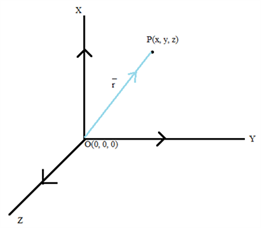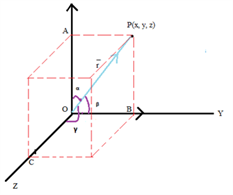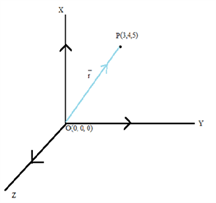Checkout JEE MAINS 2022 Question Paper Analysis : Checkout JEE MAINS 2022 Question Paper Analysis :

# Vector- Direction And Ratios

## Vector: Direction cosines

Before discussing directional cosines of a vector, let us discuss the position vector. As the name suggests, a position vector indicates the position of any point relative to any reference origin.

Consider any arbitrary point in three-dimensional space having the co-ordinates (x,y,z) as with respect to the origin O (0,0,0).The vector

$$\begin{array}{l} \overrightarrow {OP} \end{array}$$
or
$$\begin{array}{l} \overrightarrow {r} \end{array}$$
is known as the position vector and the magnitude of the position vector is given by using the distance formula as,

$$\begin{array}{l}~~~~~~~~~~\end{array}$$
$$\begin{array}{l} |\overrightarrow{OP}\end{array}$$
| =
$$\begin{array}{l}\overrightarrow{r} \end{array}$$
=
$$\begin{array}{l}\sqrt{(x-0)^2 + (y-0)^2 + (z-0)^2} \end{array}$$

$$\begin{array}{l}~~~~~~~~~~\end{array}$$
$$\begin{array}{l} \Rightarrow |\overrightarrow{OP}| \end{array}$$
=
$$\begin{array}{l}\overrightarrow{r}\end{array}$$
=
$$\begin{array}{l}\sqrt{x^2 + y^2 + z^2} \end{array}$$

### Direction cosines:

The following figure represents a vector P in space with O  as reference origin. Let the position vector make positive angle (anticlockwise direction) of α , β and γ with the positive x , y and z axis respectively. These angles are known as direction angles and on taking the cosine of these angles we get direction cosines. Taking direction cosines makes it easy to represent the direction of a vector in terms of angles with respect to the reference.From the above diagram we can easily figure out the direction cosines of the position vector as

$$\begin{array}{l}~~~~~~~~~~\end{array}$$
$$\begin{array}{l} cos \alpha\end{array}$$
=
$$\begin{array}{l}\frac {x}{|\overrightarrow{r}|}\end{array}$$

$$\begin{array}{l}~~~~~~~~~~\end{array}$$
$$\begin{array}{l} cos \beta\end{array}$$
=
$$\begin{array}{l}\frac {y}{|\overrightarrow{r}|}\end{array}$$

$$\begin{array}{l}~~~~~~~~~~\end{array}$$
$$\begin{array}{l} cos \gamma \end{array}$$
=
$$\begin{array}{l}\frac {x}{|\overrightarrow{r}|}\end{array}$$

This is the mathematical representation of cosines of the directions of a vector. The above equations can be rewritten as,

$$\begin{array}{l}~~~~~~~~~~\end{array}$$
$$\begin{array}{l} cos \alpha\end{array}$$
=
$$\begin{array}{l}\frac {x}{\sqrt{x^2 + y^2 + z^2}}\end{array}$$

$$\begin{array}{l}~~~~~~~~~~\end{array}$$
$$\begin{array}{l} cos \beta \end{array}$$
=
$$\begin{array}{l}\frac {y}{\sqrt{x^2 + y^2 + z^2}}\end{array}$$

$$\begin{array}{l}~~~~~~~~~~\end{array}$$
$$\begin{array}{l} cos \gamma\end{array}$$
=
$$\begin{array}{l} \frac {z}{\sqrt{x^2 + y^2 + z^2}}\end{array}$$

### Direction Ratios:

The co-ordinates of the point P may also be expressed as the product of the magnitude of the given vector and the cosines of direction on the three axes, i.e.

a = lr

b = mr

c = nr

where  l,m,n represent the direction cosines of the given vector on the axes X,Y,Z respectively. We can clearly see that lr,mr,nr   are in proportion to the direction cosines and these are called as the direction ratios and they are denoted by a,b,c .

$$\begin{array}{l}~~~~~~~~~~\end{array}$$

$$\begin{array}{l} \frac la \end{array}$$
=
$$\begin{array}{l} \frac {1}{\sqrt{x^2 + y^2 + x^2}} \end{array}$$

$$\begin{array}{l}~~~~~~~~~~\end{array}$$
$$\begin{array}{l} \frac mb \end{array}$$
=
$$\begin{array}{l} \frac {1}{\sqrt{x^2 + y^2 + x^2}} \end{array}$$

$$\begin{array}{l}~~~~~~~~~~\end{array}$$
$$\begin{array}{l} \frac nc \end{array}$$
=
$$\begin{array}{l} \frac {1}{\sqrt{x^2 + y^2 + x^2}} \end{array}$$

Let us now practice some examples based on the above theory,

Example: The co-ordinates of a point P(x,y,z) are (3,4,5). Determine the direction cosines and the direction ratios of the given point taking origin O (0,0,0)  as reference.

Solution: Let us represent the given point in three-dimensional Cartesian space as shownWe know that

$$\begin{array}{l}~~~~~~~~~~\end{array}$$
$$\begin{array}{l} cos \alpha \end{array}$$
=
$$\begin{array}{l}\frac {x}{\sqrt{x^2 + y^2 + z^2}}\end{array}$$

$$\begin{array}{l}~~~~~~~~~~\end{array}$$
$$\begin{array}{l} cos \beta \end{array}$$
=
$$\begin{array}{l}\frac {y}{\sqrt{x^2 + y^2 + z^2}}\end{array}$$

$$\begin{array}{l}~~~~~~~~~~\end{array}$$
$$\begin{array}{l} cos \gamma \end{array}$$
=
$$\begin{array}{l} \frac {z}{\sqrt{x^2 + y^2 + z^2}}\end{array}$$

$$\begin{array}{l} \Rightarrow \sqrt{x^2 + y^2 + z^2} \end{array}$$
=
$$\begin{array}{l} \sqrt{3^2 + 4^2 + 5^2} \end{array}$$

$$\begin{array}{l} \Rightarrow \sqrt{x^2 + y^2 + z^2} \end{array}$$
=
$$\begin{array}{l} \sqrt{50} = 5 \sqrt{2} \end{array}$$

Therefore, we can say that

l = cos α =

$$\begin{array}{l} \frac {3}{5\sqrt{2}} \end{array}$$

m = cos β =

$$\begin{array}{l} \frac {4}{5\sqrt{2}} \end{array}$$

n = cos γ =

$$\begin{array}{l} \frac {5}{5\sqrt{2}} = \frac {1}{\sqrt{2}} \end{array}$$

The direction ratios of the given vector will be 3:4:5 .

⇒ a = 3

⇒ b = 4

⇒ c = 5

From the above example, it can be also be figured out that

$$\begin{array}{l} l^2 + m^2 + n^2 \end{array}$$
=
$$\begin{array}{l} (\frac{3}{5\sqrt{2}})^2 + (\frac{4}{5\sqrt{2}})^2 + (\frac{5}{5\sqrt{2}})^2 \end{array}$$
= 1

But

$$\begin{array}{l} a^2 + b^2 + c^2 \ne 1 \end{array}$$
<

Visit us online at www.byjus.com to know more about vectors.